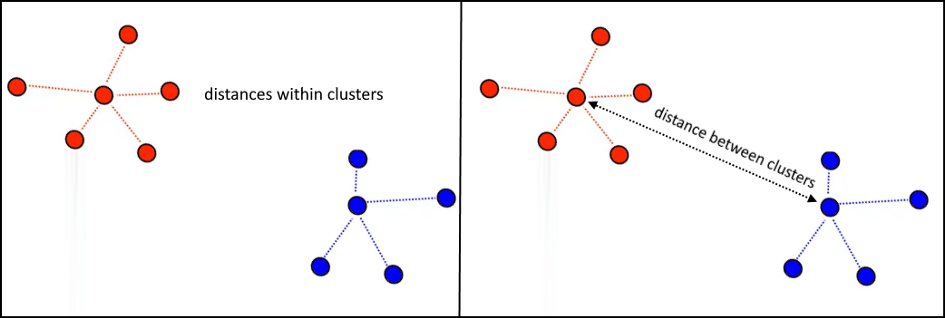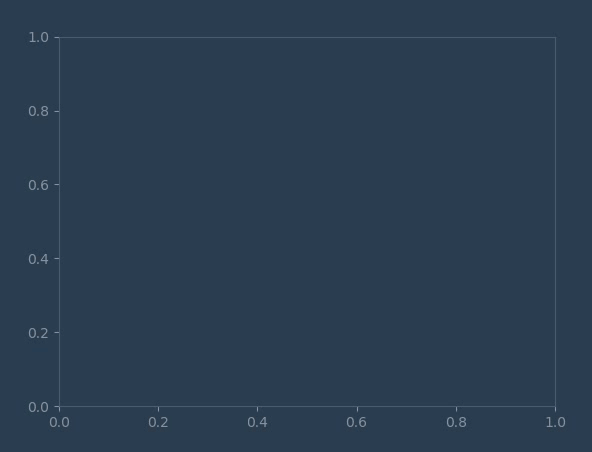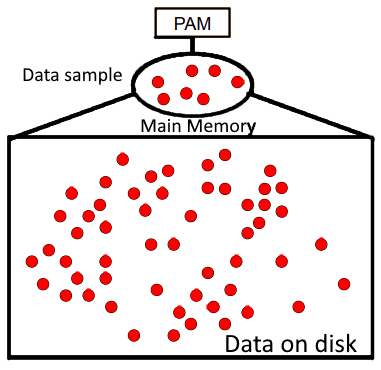top of page
Search

# Clustering-Types of Clustering and Clustering Algorithms used in Data Science and Data Mining

Updated: Mar 27

Clustering is a technique that involves grouping a set of data points into subsets or clusters based on their similarity. The objective of clustering is to identify patterns or structures in the data by grouping similar objects together, while also keeping dissimilar objects apart. Clustering algorithms can be used for a variety of applications, including customer segmentation, image recognition, anomaly detection, and data compression, among others. There are several types of clustering algorithms, including hierarchical clustering, k-means clustering, and density-based clustering, each with its own strengths and weaknesses.## Types of Clustering:

Clustering can be divided into two subgroups :

• Hard Clustering: In hard clustering, each data point is assigned to exactly one cluster. This means that the data points in each cluster are similar to each other and dissimilar to those in other clusters. Hard clustering algorithms include k-means clustering, hierarchical clustering, and partitioning around medoids (PAM).

• Soft Clustering: In soft clustering, each data point can belong to multiple clusters with different degrees of membership or similarity. This means that the data points in each cluster are similar to each other, but they may also have some similarities with the data points in other clusters. Soft clustering algorithms include fuzzy clustering, where data points have membership degrees ranging between 0 and 1, and probabilistic clustering, such as the Gaussian mixture model (GMM), where data points are assigned to clusters based on the probability of belonging to each cluster.

## Clustering Algorithms used in Data Science and Data Mining

Clustering algorithms can be classified based on the purpose they are trying to achieve. Therefore, exists two types of Clustering techniques based on this criterion:

1. Monothetic: Exists some common properties between cluster members(e.g., 25% of patients show side effects due to vaccine A): the data are divided on values generated by a single feature.

2. Polythetic: Exists some degree of similarity between cluster members without having a common property(e.g., dissimilarity measure): the data are divided on values generated by all features.

Based on the clustering analysis technique being used, each cluster presents a centroid, a single observation representing the centre of the data samples, and a boundary limit. The following illustration represents some common categories of clustering algorithms.

### Clustering Algorithm

1. Centroid Based Clustering

• K-means

• k-means ++

• K-means l l

• Fuzzy C-means

• k-models, PAM

• Medians

• K-modes

• K-Prototypes

• CLARA

• CLARANS

2. Distribution Based Clustering

• GMM

• EM

• DMM

3. Density Based Clustering

• DBSCAN

• DENCLUE

• OPTICS

## Centroid Based Clustering

These are iterative clustering algorithms in which the notion of similarity is derived by the closeness of a data point to the centroid of the clusters. K-Means clustering algorithm is a popular algorithm that falls into this category. In these models, the no. of clusters required at the end have to be mentioned beforehand, which makes it important to have prior knowledge of the dataset. These models run iteratively to find the local optima.

### 1. K-means

the goal of the k-means algorithm is to maximize the distance between each pair of clusters’ centers and minimize the distance between observations within each cluster (e.g., minimize the sum of squared error, SSE, within a cluster.).k- means clustering works well if the following conditions are met:

• The distribution’s variance of each attribute is spherical.

• Clusters are linearly separable.

• Clusters have similar numbers of observations(closer size.).

• Variables present the same variance.

• The learning curve is relatively steep.

• Widely implemented by a variety of packages(Stats package in R, scikit-learn in python…)

• Fast convergence for clustering small datasets.

• Easy to implement.

• Computationally expensive for large datasets(k becomes large.).

• Sometimes, it is difficult to choose an initial value for the number of clusters(k).

• Doesn’t guarantee to converge to a global minimum. It is sensitive to the centroids’ initialization. Different setups may lead to different results.

• Strong sensitivity to outliers.

### 2. k-means ++

The idea behind k-means++ is that it tries to spread out the centres while allocating a new centre per iteration. Therefore, the algorithm starts by randomly(uniform) picking an initial centre from the dataset, which means that all points have an equal probability of getting selected. Then, the distance squared from each data point to the previously chosen centre is computed. After that, it computes the probability for each data point by simply dividing the distance by the total distances. Further, assign a new cluster centre to the point that has the highest probability or the highest distance. In other words, the likelihood of a data object being the centre of a new cluster is proportional to the distance squared.### 3. K-means l l

K-means parallel is another sufficient technique that updates the distribution of the samples less frequently after each iteration. It introduces an oversampling factor (L ~ order of k., e.g., k, k/2, …) to the k-means algorithm. Using that factor, it will make the algorithm converge much faster for larger datasets.1. Scales well for large datasets. runtime ~log(k)

2. Faster than K means ++ because it simples L centroids per iteration

1. It can lead to over-sampling or under-sampling based on the value of L

### 4. Fuzzy C-means

The term fuzzy was used to stress the fact that there are various shades of clusters(e.g., disjoint, non disjoints…) that are allowed to form where a data point can exist in one or more clusters. For instance, the color orange is a mixture of red and yellow colors, which means that it belongs to each color group to some degree.A membership degree function is used to measure the degree of belonging of a data point to each cluster. It describes the probability that a data point belongs to a certain cluster.

1. Better results for overlapped data in contrast to K-means

2. Low time Complexity

3. Convergence is guaranteed.

1. Sensitive to the initial value of K and P

2. Sensitive to outliers

### 5. k-models, PAM(Partitioning Around Medoids)

A modified version of the k-means algorithm where a medoid represents a data point with the lowest average dissimilarity among all points within a cluster. The purpose is to minimize the overall cost for each cluster. Unlike k-means, it uses a medoid as a metric to reassign the centroid of each cluster. Medoids are less sensitive to outliers. These medoids are actual observations from the dataset and not computed points(mean value) like in the case of k-means. It is preferred to use the manhattan distance as a metric because it is less sensitive to outliers.

1. More robust than k-means in the presence of outliers(Less influenced by outliers.)

2. It can be Implemented Easily.

3. It converges in a fixed number of iterations.

4. It works effectively with a small dataset.

1. It doesn’t scale well for a large dataset.

2. The computational complexity is quite expensive.

3. The parameter k needs to be initialized to a certain value.

4. Doesn’t guarantee to converge to a global minimum. It is sensitive to the centroids’ initialization. Different setups lead to different results.

5. Works only on numerical data.

### 6. Medians

A modified version of the k-means algorithm uses the median which represents the middle point where other observations are evenly distributed around it. A median is less sensitive to outliers than a mean.Additionally, it uses the Manhattan distance as a metric for computing distances between observations.

### 7. K-modes

Since K-means handles only numerical data attributes, a modified version of the k-means algorithm has been developed to cluster categorical data. The mode replaces the mean in each cluster. However, someone could come with the idea of mapping between categorical and numerical attributes and then clustering using k-means. This could sometimes work on a small dimensional dataset. But, mapping between two different types of attributes cannot guarantee a high-quality clustering for high dimensional data. Therefore, it is recommended to use k-modes when clustering categorical data attributes.

One of the dissimilarity measures used in k-modes is the cosine dissimilarity measure, a frequency-based method that computes the distance between two observations(e.g., the distance between two sentences or two documents).

1. Able to cluster categorical data attributes

2. It converges faster than K-prototypes.

1. Computationally expensive for large datasets(k becomes large.).

2. Sometimes, it is difficult to choose the right initial value for the number of clusters(k).

3. Doesn’t guarantee to converge to a global minimum. It is sensitive to the centroids’ initialization. Different setups may lead to different results.

4. Efficiency depends on the dissimilarity measure used by the algorithm(e.g. Spearman correlation, cosine distance…).

5. Additional variable is added to the algorithm(𝛾) that controls the weight of the distance from each observation to their clusters’ centers.

### 8. K-Prototypes

This method works on a mixture of numerical and categorical data attributes. This algorithm can be thought of as a composition between k-means and k-modes algorithms.

Using this algorithm, each data point has a weight being a part of numerical and categorical clusters. Moreover, each type of observation can be treated in a separate fashion where centroids play the role of an attractor in each type of cluster. The membership to a given data point can be controlled using a fuzzy membership function aij like in FCM.1. Ability to cluster mixed types of attributes.

2. Converge in a reasonable number of iterations.

1. Different dissimilarity measures can lead to different outcomes.

2. Sensitive to the initial values of k and 𝛾.

3. Doesn’t guarantee to converge to a global minimum. It is sensitive to the medoids’ initialization. Different setups may lead to different results.

4. Efficiency depends on the dissimilarity measure used by the algorithm(e.g. Spearman correlation, cosine distance…).

5. Slower than k-modes in the case of clustering categorical data.

### 9. CLARA(Clustering Large Applications)

It is a sample-based method that randomly selects a small subset of data points instead of considering the whole observations, which means that it works well on a large dataset. Moreover, k medoids are chosen from the previously selected sample. This will help towards improving the scalability of PAM(reduce computing time and memory allocation problems). It works sequentially on different batches of the dataset to find the most optimal result.1. Ability to handle large datasets.

2. Reduce the computation time while dealing with a large data set.

3. Ability to handle outliers.

Drawbacks.

1. Efficiency is influenced by the value of k and the sample size.

2. The quality of clustering depends on the quality of the sampling method being used.

3. Hard to implement.

### 10. CLARANS(Clustering Large Applications based on RANdomized Search)

It is an extension to k-medoid used in data mining to cluster large datasets.1. More effective than PAM and CLARA on large datasets.

2. Ability to handle outliers.

Drawbacks.

1. Hard to implement.

2. The quality of clustering depends on the quality of the sampling method being used.

## Distributions Based Clustering

These clustering models are based on the notion of how probable is it that all data points in the cluster belong to the same distribution (For example: Normal, Gaussian). These models often suffer from overfitting. A popular example of these models is the Expectation-maximization algorithm which uses multivariate normal distributions.

### 1. GMM(Gaussian Mixture Model)

In 2-d variables space, a gaussian distribution is a bivariate normal distribution constructed using two random variables having a normal distribution, each parameterized by its mean and standard deviation.The Gaussian Mixture Model is a semi-parametric model (finite number of parameters that increases with data.) used as a soft clustering algorithm where each cluster corresponds to a generative model that aims to discover the parameters of a probability distribution (e.g., mean, covariance, density function…) for a given cluster(its own probability distribution governs each cluster). The process of learning is to fit a gaussian model to the data points. The Gaussian Mixture model assumes that the clusters are distributed in a normal distribution in n-dimensional space.

### 2. EM (expectation-Maximization in Context of Clustering)

It is a well-known algorithm for fitting mixture distributions that aims to estimate the parameters of a given distribution using the maximum likelihood principle(finding the optimum values) when some of the data points are not available(e.g., Unknown parameters, latent values…). In the context of GMM, The intuition is to randomly place k gaussian models in space and compute the degree of belonging of each data point to a certain gaussian model. Unlike hard clustering(e.g., k-means), the method computes the probabilities for each point to be a member of a certain cluster. Further, these values are used to reestimate the cluster parameters(e.g., mean, covariance)to fit the points assigned for each cluster.

EM is widely used to solve problems such as the “hidden-data” problems, the Hidden Markov Models, where there is a sequence of latent variables that depends on the state of the previously hidden variable.

### 3. DMM (Dirichlet Mixtures Models)The Dirichlet process is a stochastic process that produces a distribution over a discrete distribution(probability measures) used for defining Bayesian non-parametric(unfixed set of parameters. e.g., ~ infinite number of parameters.) models. The Dirichlet distribution is a continuous multivariate density function parameterized by a concentration/precision parameter/vector (α₁, …, αₖ) with positive components and a base distribution H: DP(α, H). It is like a Beta distribution(e.g., Coinflip) for more than two outcomes.

## Density Based Clustering

These models search the data space for areas of varied density of data points in the data space. It isolates various different density regions and assign the data points within these regions in the same cluster. Popular examples of density models are DBSCAN and OPTICS.

### 1. DBSCAN: Density-Based Spatial Clustering of Applications with Noise.

It is by far the most popular density-based clustering algorithm with more than 41k citations on Google Scholar. The central idea is to partition the observations into 3 types of points group:

1. Core points: There are more than minPts points in the ε-neighborhood.

2. Boundary points: Fewer than minPts within ε but in the neighborhood of a core point.

3. Noise or Outlier points: All remaining points: Not a core point, and not close enough to be reachable from a core point.

As the name suggests, this algorithm differs from the previous one by adapting the values of Eps and MinPts on behalf of the density distribution for each cluster. It automatically finds proper Eps and MinPts values.

It starts by randomly choosing a value for Eps. Then, it runs DBSCAN on the dataset, and if it fails to find a cluster, it increases the value of Eps by 0.5. When the algorithm finds a cluster(10% of similar data), it excludes the cluster from the dataset. And, the algorithm keeps increasing the value of Eps to find the next cluster. Once the algorithm successfully finishes scanning around 95% of the data, the remaining data points will be declared outliers.

### 3. DENCLUE ( DENsity- based CLUstEring)

DENCLUE applies the kernel density estimation method to estimate a random variable’s undefined probability density function that generates the data sample. The estimation is based on a kernel density function(e.g., Gaussian density function.) representing the distribution of each data point. Then, the kernel density estimate of all the previous functions is computed by summing them up(or integral). A kernel is a mathematical function that models the influence between data points and their neighbors.

### 4. OPTICS: Ordering Points To Identify Clustering Structure.

Since the performance of DBSCAN depends on its parameters setup, Optics has extended DBSCAN, makes it less sensitive to parameter setting, and finding structures among clusters. The intuition is that higher density regions will be processed first before the lower ones based on two parameters:

1. Core distance: The smallest radius eps that contains at least MinPts observations.

2. Reachability distance: The minimum distance that makes two observations density-reachable from each other.

1. Discovery of underlying structures: Clustering can help identify groups of similar data points that may represent underlying structures or patterns in the data, which can then be used for further analysis and insight generation.

2. Scalability: Clustering algorithms can be applied to large and complex datasets with a high number of data points and dimensions.

3. Flexibility: Clustering can be used with a wide range of data types, including numerical, categorical, and textual data.

4. Unsupervised learning: Clustering is an unsupervised learning technique, which means that it does not require labelled data and can be used to identify hidden patterns or structures in the data.